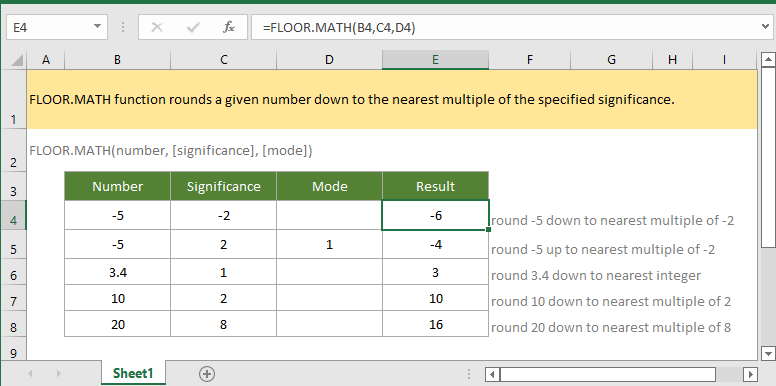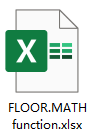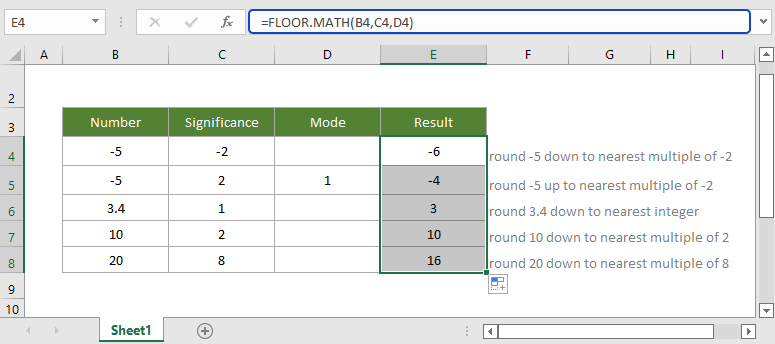## Excel FLOOR.MATH Function### Description

The ExcelFLOOR.MATH function rounds a given number down to the nearest integer or nearest multiple of the specified significance.

Differ from FLOOR function, the argument significance of FLOOR.MATH function can be omitted (if it is omitted, the number will be rounded down to the nearest integer, for example, FLOOR.MATH(4.5)=4), and the argument mode can be used to be choose the direction to round negative numbers.

### Syntax and arguments

Formula syntax

FLOOR.MATH(number, [significance], [mode])

Arguments

 Number: Required, the number that used to round. Significance: Optional, the multiple to which used to round. Default is 1 if it is omitted. Note the arithmetic sign of significance is ignored. Mode: Optional, a numeric value that decides round number down or up when number is negative. -when mode is 0 or omitted, a negative number is rounded down (away from zero), for example, FLOOR.MATH(-5,2)=-6. -when mode is other numeric value, a negative number is rounded up (toward zero), for example, FLOOR.MATH(-5,2,1)=-4.

Return Value

The FLOOR.MATH function returns to a numeric value.

Errors

#VALUE! error value occurs when the anyone of arguments is not a numeric value.

Remarks

If the argument number is an exact multiple of significance, FLOOR.MATH function returns to the number itself, for example, FLOOR.MATH(10,2)=10.

Version

Excel 2013 or later

### Usage and Examples

Example: basic usage

In table B4:D8 which contains the numbers, specified significances and modes, you can use FLOOR.MARH function to round numbers down and get the nearest integers or multiples as this:

=FLOOR.MATH(B4,C4,D4)

Press Enter key to get the first result, then drag autofill handle down to get all results.#### Relative Functions:

• Excel FACT Function
The FACT function eturns the factorial of a given number. For example, FACT(5)=120, equivalent to 5 x 4 x 3 x 2 x 1.

• Excel FACTDOUBLE Function
The FACTDOUBLE function returns the double factorial of a given number. For example, FACTDOUBLE(5)=15, equivalent 5 x 3 x 1.

• Excel FLOOR Function
The FLOOR function rounds a given number down to the nearest multiple of the specified significance.

The RECEIVED function returns the amount received at maturity for a fully invested security.

### The Best Office Productivity Tools

#### Kutools for Excel - Helps You To Stand Out From Crowd

 Popular Features: Find, Highlight or Identify Duplicates  |  Delete Blank Rows  |  Combine Columns or Cells without Losing Data  |  Round without Formula ... Super VLookup: Multiple Criteria  |  Multiple Value  |  Across Multi-Sheets  |  Fuzzy Lookup... Adv. Drop-down List: Easy Drop Down List  |  Dependent Drop Down List  |  Multi-select Drop Down List... Column Manager: Add a Specific Number of Columns  |  Move Columns  |  Toggle Visibility Status of Hidden Columns  |  Compare Columns to Select Same & Different Cells ... Featured Features: Grid Focus  |  Design View  |  Big Formula Bar  |  Workbook & Sheet Manager | Resource Library (Auto Text)  |  Date Picker  |  Combine Worksheets  |  Encrypt/Decrypt Cells  |  Send Emails by List  |  Super Filter  |  Special Filter (filter bold/italic/strikethrough...) ... Top 15 Toolsets:  12 Text Tools (Add Text, Remove Characters ...)  |  50+ Chart Types (Gantt Chart ...)  |  40+ Practical Formulas (Calculate age based on birthday ...)  |  19 Insertion Tools (Insert QR Code, Insert Picture from Path ...)  |  12 Conversion Tools (Numbers to Words, Currency Conversion ...)  |  7 Merge & Split Tools (Advanced Combine Rows, Split Excel Cells ...)  |  ... and more

Kutools for Excel Boasts Over 300 Features, Ensuring That What You Need is Just A Click Away...#### Office Tab - Enable Tabbed Reading and Editing in Microsoft Office (include Excel)

• One second to switch between dozens of open documents!
• Reduce hundreds of mouse clicks for you every day, say goodbye to mouse hand.
• Increases your productivity by 50% when viewing and editing multiple documents.
• Brings Efficient Tabs to Office (include Excel), Just Like Chrome, Edge and Firefox.